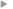## Modelling spatially correlated observation errors in variational data assimilation using a diffusion operator on an unstructured mesh

#### Guillet, Oliver ; Weaver, Anthony T. ; Vasseur, Xavier ; Michel, Yann ; Gratton, Serge ; Gürol, Selime

Année de publication
2019
Résumé
<p align="justify">We propose a method for representing spatially correlated observation errors in variational data assimilation. The method is based on the numerical solution of a diffusion equation, a technique commonly used for representing spatially correlated background errors. The discretization of the pseudo-time derivative of the diffusion equation is done implicitly using a backward Euler scheme. The solution of the resulting elliptic equation can be interpreted as a correlation operator whose kernel is a correlation function from the Matérn family. In order to account for the possibly heterogeneous distribution of observations, a spatial discretization technique based on the finite element method (FEM) is chosen where the observation locations are used to define the nodes of an unstructured mesh on which the diffusion equation is solved. By construction, the method leads to a convenient operator for the inverse of the observation-error correlation matrix, which is an important requirement when applying it with standard minimization algorithms in variational data assimilation. Previous studies have shown that spatially correlated observation errors can also be accounted for by assimilating the observations together with their directional derivatives up to arbitrary order. In the continuous framework, we show that the two approaches are formally equivalent for certain parameter specifications. The FEM provides an appropriate framework for evaluating the derivatives numerically, especially when the observations are heterogeneously distributed. Numerical experiments are performed using a realistic data distribution from the Spinning Enhanced Visible and InfraRed Imager (SEVIRI). Correlations obtained with the FEM-discretized diffusion operator are compared with those obtained using the analytical Matérn correlation model. The method is shown to produce an accurate representation of the target Matérn function in regions where the data are densely distributed. The presence of large gaps in the data distribution degrades the quality of the mesh and leads to numerical errors in the representation of the Matérn function. Strategies to improve the accuracy of the method in the presence of such gaps are discussed.</p>
Texte intégralAccès à la notice sur le site du portail documentaire de Météo-FranceListe complète des notices publiques